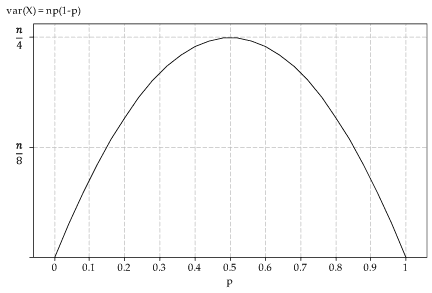##### Exercise 1
1. Let $$X$$ be the number of winning wrappers in one week (7 days). Then $$X \stackrel{\mathrm{d}}{=} \mathrm{Bi}(7,\frac{1}{6})$$.
2. $$\Pr(X = 0) = \bigl(\dfrac{5}{6}\bigr)^7 \approx 0.279$$.
3. The purchases are assumed to be independent, and hence the outcome of the first six days is irrelevant. The probability of a winning wrapper on any given day (including on the seventh day) is $$\dfrac{1}{6}$$.
4. Let $$Y$$ be the number of winning wrappers in six weeks (42 days). Then $$Y \stackrel{\mathrm{d}}{=} \mathrm{Bi}(42,\frac{1}{6})$$. Hence, \begin{align*} \Pr(Y \geq 3) &= 1 - \Pr(Y \leq 2) \\ &= 1 - \bigl[\Pr(Y=0) + \Pr(Y=1) + \Pr(Y=2)\bigr] \\ &= 1 - \bigl[\bigl(\tfrac{5}{6}\bigr)^{42} + 42 \times \tfrac{1}{6} \times \bigl(\tfrac{5}{6}\bigr)^{41} + 861 \times \bigl(\tfrac{1}{6}\bigr)^2 \times \bigl(\tfrac{5}{6}\bigr)^{40}\bigr] \\ &\approx 1 - \bigl[0.0005 + 0.0040 + 0.0163\bigr] \approx 0.979. \end{align*} This could also be calculated with the help of the $$\sf \text{BINOM.DIST}$$ function in Excel.
5. Let $$U$$ be the number of winning wrappers in $$n$$ purchases. Then $$U \stackrel{\mathrm{d}}{=} \mathrm{Bi}(n,\frac{1}{6})$$ and so \begin{align*} \Pr(U \geq 1) \geq 0.95 \ &\iff\ 1 - \Pr(U = 0) \geq 0.95 \\ &\iff\ \Pr(U = 0) \leq 0.05 \\ &\iff\ \bigl(\tfrac{5}{6}\bigr)^n \leq 0.05 \\ &\iff\ n\,\log_e\bigl(\tfrac{5}{6}\bigr) \leq \log_e(0.05) \\ &\iff\ n \geq \dfrac{\log_e(0.05)}{\log_e\bigl(\tfrac{5}{6}\bigr)} \approx 16.431. \end{align*} Hence, he needs to purchase a bar every day for 17 days.
##### Exercise 2
1. Binomial.
2. Not binomial. The weather from one day to the next is not independent. Even though, for example, the chance of rain on a day in March may be 0.1 based on long-term records for the city, the days in a particular fortnight are not independent.
3. Not binomial. When sampling without replacement from a small population of tickets, the chance of a 'success' (in this case, a blue ticket) changes with each ticket drawn.
4. Not binomial. There is likely to be clustering within classes; the students within a class are not independent from each other.
5. Binomial.
##### Exercise 3

This problem is like that of Casey and the winning wrappers. Let $$X$$ be the number of sampled quadrats containing the species of interest. We assume that $$X \stackrel{\mathrm{d}}{=} \mathrm{Bi}(n,k)$$. We want $$\Pr(X \geq 1) \geq 0.9$$, since we only need to observe the species in one quadrat to know that it is present in the forest. We have

\begin{align*} \Pr(X \geq 1) \geq 0.9 \ &\iff\ \Pr(X = 0) \leq 0.1 \\ &\iff\ (1-k)^n \leq 0.1 \\ &\iff\ n \geq \dfrac{\log_e(0.1)}{\log_e(1-k)}. \end{align*}

For example: if $$k = 0.1$$, we need $$n \geq 22$$; if $$k=0.05$$, we need $$n \geq 45$$; if $$k=0.01$$, we need $$n \geq 230$$.

##### Exercise 4
1. $$p$$ 0.01 0.05 0.2 0.35 0.5 0.65 0.8 0.95 0.99
$$\mu_X$$ 0.2 1 4 7 10 13 16 19 19.8
$$\mathrm{sd}(X)$$ 0.445 0.975 1.789 2.133 2.236 2.133 1.789 0.975 0.445

2. The standard deviation is smallest when $$|p-0.5|$$ is largest; in this set of distributions, when $$p=0.01$$ and $$p = 0.99$$. The standard deviation is largest when $$p = 0.5$$.
##### Exercise 5
1. The graph of the variance of $$X$$ as a function of $$p$$ is as follows.Figure 2: Variance of $$X$$ as a function of $$p$$, where $$X \stackrel{\mathrm{d}}{=} \mathrm{Bi}(n,p)$$.

2. The variance function is $$f(p) = np(1-p)$$. So $$f'(p) = n(1-2p)$$. Hence $$f'(p) = 0$$ when $$p = 0.5$$, and this is clearly a maximum.
3. When $$p = 0.5$$, we have $$\mathrm{var}(X) = \dfrac{n}{4}$$ and $$\mathrm{sd}(X) = \dfrac{\sqrt{n}}{2}$$.
##### Exercise 6

Yes, it is true that, as $$n$$ tends to infinity, the largest value of $$p_X(x)$$ tends to zero. We will not prove this explicitly, but make the following two observations.

• First consider the case where $$p = 0.5$$ and $$n$$ is even; let $$n=2m$$. The largest value of $$p_X(x)$$ occurs when $$x = \dfrac{n}{2} = m$$ (see figure 2, for example). This probability is given by $p_X(m) = \dbinom{2m}{m}\, \dfrac{1}{4^m}.$ This tends to zero as $$m$$ tends to infinity. Proving this fact is beyond the scope of the curriculum (it can be done using Stirling's formula, for example), but evaluating the probabilities for large values of $$m$$ will suggest that it is true:
• for $$m=1000$$, $$p_X(m) \approx 0.0178$$
• for $$m=10\ 000$$, $$p_X(m) \approx 0.0056$$
• for $$m=100\ 000$$, $$p_X(m) \approx 0.0018$$
• for $$m=1\ 000\ 000$$, $$p_X(m) \approx 0.0006$$.
• In general, for $$X \stackrel{\mathrm{d}}{=} \mathrm{Bi}(n,p)$$, we have $$\mathrm{sd}(X) = \sqrt{np(1-p)}$$. As $$n$$ tends to infinity, the standard deviation grows larger and larger, which means that the distribution is more and more spread out, leading to the largest probability gradually diminishing in size.